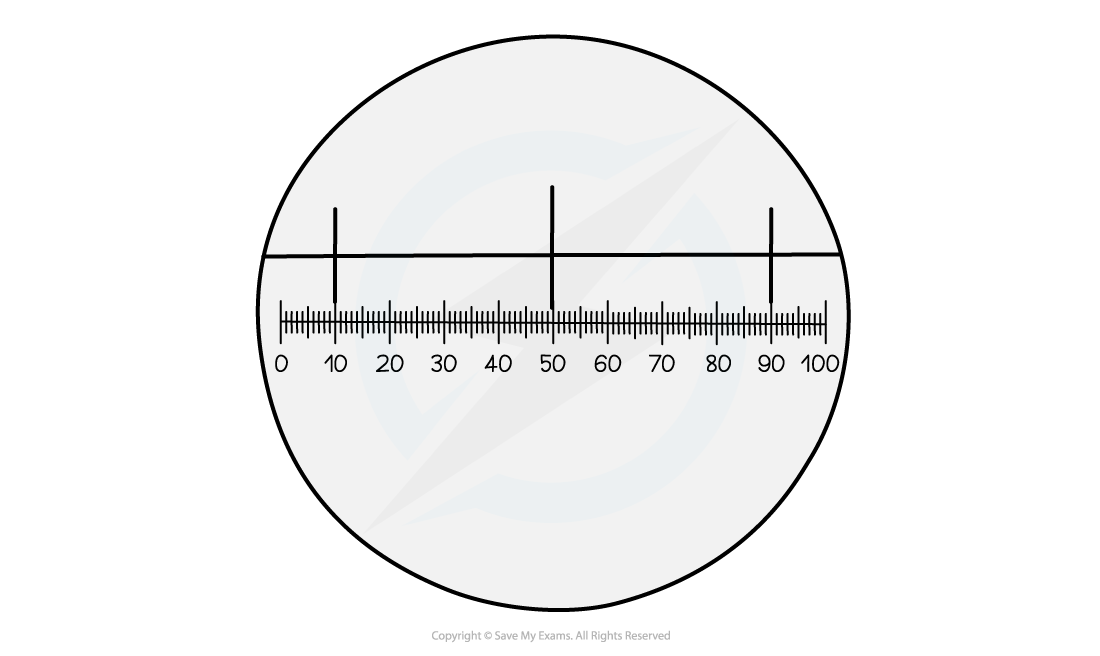# CIE A Level Biology复习笔记1.1.3 Eyepiece Graticules & Stage Micrometers

### Eyepiece Graticules & Stage Micrometers

• An eyepiece graticule and stage micrometer are used to measure the size of the object when viewed under a microscope
• Each microscope can vary slightly so needs to be calibrated when used
• The calibration is done with a stage micrometer, this is a slide with a very accurate scale in micrometres (µm), it is usually in 10 µm divisions, so 1 mm divided into 100 divisions
• The eyepiece graticule is a disc placed in the eyepiece with 100 divisions, this has no scale
• To know what the divisions equal at each magnification the eyepiece graticule is calibrated to the stage micrometer at each magnification

#### Using stage micrometer & eyepiece graticule• In the diagram, the stage micrometer has three lines each 10 µm apart
• Each 10 µm division has 40 eyepiece graticule divisions
• 40 graticule divisions = 10 µm

1 graticule division = number of micrometres ÷ number of graticule division

• 1 graticule division = 10 ÷ 40 = 0.25 µm this is the magnification factor
• The specimen slide would be used to replace the stage micrometer and the eyepiece graticule at the same magnification would be used to measure the length of the object
• The number of graticule divisions can then be multiplied by the magnification factor:

graticule divisions x magnification factor = measurement (µm)

#### Exam Tip

The calculations involving stage micrometers and eyepiece graticules are often seen in exam questions, so make sure that you are comfortable with how to calibrate the graticule and calculate the length of an object on the slide.